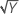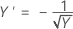# Methods and formulas for model information in Analyze Response Surface Design

Select the method or formula of your choice.

## Design matrix

Minitab generates a design matrix for each design. The first column is a column of one's for the constant term. If the design was blocked into k blocks, there are (k − 1) columns for blocks. Minitab uses the same method of coding blocks as in Factorial Models. This is followed by one column for each main effect. Terms with categorical factors can have more than one column. If the model has squared terms, there is one column for each squared term. The column for a squared term is the product of the corresponding factor with itself. If the model has interaction terms, then there is one column for each interaction term. Interactions that include categorical factors can have more than one column. The column for an interaction term is the product of the two columns that are crossed.

If Minitab removes some terms because the data cannot support them, these terms are not in the stored design matrix. The columns stored match the coefficients that are displayed.

## Coefficient (Coef)

In matrix terms, the formula that calculates the vector of coefficients in the model is:

TermDescription
Xdesign matrix
Yresponse vector

## Box-Cox transformation

Box-Cox transformation selects lambda values, as shown below, which minimize the residual sum of squares. The resulting transformation is Y λ when λ ≠ 0 and ln(Y) when λ = 0. When λ < 0, Minitab also multiplies the transformed response by −1 to maintain the order from the untransformed response.

Minitab searches for an optimal value between −2 and 2. Values that fall outside of this interval might not result in a better fit.

Here are some common transformations where Y′ is the transform of the data Y:

Lambda (λ) value Transformation
λ = 2 Y′ = Y 2
λ = 0.5 Y′ =λ = 0 Y′ = ln(Y )
λ = −0.5λ = −1 Y′ = −1 / Y
By using this site you agree to the use of cookies for analytics and personalized content.  Read our policy